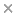BaseSpace
Correlation Engine 2.0Search sequence regions

###### filter terms:
Sizes of these terms reflect their relevance to your search.

The constant surface tension assumption of the Classical Nucleation Theory (CNT) is known to be flawed. In order to probe beyond this limitation, we consider a microscopic, two-dimensional Lattice-Gas Automata (LGA) model of nucleation in a supersaturated system, with model input parameters E(ss) (solid particle-to-solid particle bonding energy), E(sw) (solid particle-to-water bonding energy), η (next-to-nearest-neighbor bonding coefficient in solid phase), and C(in) (initial solute concentration). The LGA method has the advantages of easy implementation, low memory requirements, and fast computation speed. Analytical results for the system's concentration and the crystal radius as functions of time are derived and the former is fit to the simulation data in order to determine the equilibrium concentration. The "Mean First-Passage Time" technique is used to obtain the nucleation rate and critical nucleus size from the simulation data. The nucleation rate and supersaturation data are evaluated using a modification to the CNT that incorporates a two-dimensional radius-dependent surface tension term. The Tolman parameter, δ, which controls the radius dependence of the surface tension, decreases (increases) as a function of the magnitude of E(ss) (E(sw)), at fixed values of η and E(sw) (E(ss)). On the other hand, δ increases as η increases while E(ss) and E(sw) are held constant. The constant surface tension term of the CNT, Σ(0), increases (decreases) with increasing magnitudes of E(ss) (E(sw)) at fixed values of E(sw) (E(ss)) and increases as η is increased. Σ(0) increases linearly as a function of the change in energy during an attachment or detachment reaction, |ΔE|, however, with a slope less than that predicted for a crystal that is uniformly packed at maximum density. These results indicate an increase in the radius-dependent surface tension, Σ, with respect to increasing magnitude of the difference between E(ss) and E(sw).

### Citation

Joseph Hickey, Ivan L'Heureux. Classical nucleation theory with a radius-dependent surface tension: a two-dimensional lattice-gas automata model. Physical review. E, Statistical, nonlinear, and soft matter physics. 2013 Feb;87(2):022406

PMID: 23496525

View Full Text